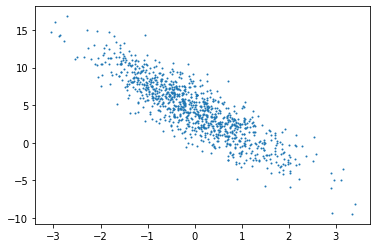# 家乐的深度学习笔记「3」 - 线性回归

2019/04/10 10:10

[TOC]

## 线性回归

### 基本要素

#### 损失函数

$w_1^, w_2^, b^* = \operatorname*{argmin}_{w_1, w_2, b}\ \ell(w_1, w_2, b)$

2020-03-29：使用训练数据集中所有样本的误差的平均来衡量模型预测的质量，表示刚想通这个概念，刚开始把小批量的概念混在一起了，但是，使用小批量的误差来反向传播更新模型的梯度，而不是整体所有样本。

# 广义上讲，当数据样本数为$n$，特征数为$d$时，线性回归的矢量计算表达式为 $\boldsymbol{\hat{y}} = \boldsymbol{X} \boldsymbol{w} + b$ 其中模型输出$\boldsymbol{\hat{y}} \in \mathbb{R}^{n \times 1}$， 批量数据样本特征$\boldsymbol{X} \in \mathbb{R}^{n \times d}$，权重$\boldsymbol{w} \in \mathbb{R}^{d \times 1}$， 偏差$b \in \mathbb{R}$。相应地，批量数据样本标签$\boldsymbol{y} \in \mathbb{R}^{n \times 1}$。设模型参数$\boldsymbol{\theta} = [w_1, w_2, b]^\top$，我们可以重写损失函数为 $\ell(\boldsymbol{\theta})=\frac{1}{2n}(\boldsymbol{\hat{y}}-\boldsymbol{y})^\top(\boldsymbol{\hat{y}}-\boldsymbol{y})$ 小批量随机梯度下降的迭代步骤将相应地改写为 $\boldsymbol{\theta} \leftarrow \boldsymbol{\theta} - \frac{\eta}{|\mathcal{B}|} \sum_{i \in \mathcal{B}} \nabla_{\boldsymbol{\theta}} \ell^{(i)}(\boldsymbol{\theta})$ 其中梯度是损失有关3个为标量的模型参数的偏导数组成的向量： $\nabla_{\boldsymbol{\theta}} \ell^{(i)}(\boldsymbol{\theta})= \begin{bmatrix} \frac{ \partial \ell^{(i)}(w_1, w_2, b) }{\partial w_1} \ \frac{ \partial \ell^{(i)}(w_1, w_2, b) }{\partial w_2} \ \frac{ \partial \ell^{(i)}(w_1, w_2, b) }{\partial b} \end{bmatrix} # \begin{bmatrix} x_1^{(i)} (x_1^{(i)} w_1 + x_2^{(i)} w_2 + b - y^{(i)}) \ x_2^{(i)} (x_1^{(i)} w_1 + x_2^{(i)} w_2 + b - y^{(i)}) \ x_1^{(i)} w_1 + x_2^{(i)} w_2 + b - y^{(i)} \end{bmatrix} \begin{bmatrix} x_1^{(i)} \ x_2^{(i)} \ 1 \end{bmatrix} (\hat{y}^{(i)} - y^{(i)})$

### 从零实现

from mxnet import gpu, nd, autograd
import matplotlib.pyplot as plt
import random


random.seed(233)


#### 生成数据集

ctx = gpu(3)

num_examples = 1000
num_inputs = 2
true_w = [2, -3.4]
true_b = 4.2

features = nd.random.normal(scale=1, shape=(num_examples, num_inputs), ctx=ctx)
labels = true_w * features[:, 0] + true_w * features[:, 1] + true_b
labels += nd.random.normal(scale=0.01, shape=labels.shape, ctx=ctx)


features[:5], labels[:5]

(
[[ 0.34113222  0.76659095]
[ 0.6508758  -1.5585263 ]
[ 1.9786316  -0.8465744 ]
[-0.05428567  0.64376295]
[-1.7093595   0.12594718]]
<NDArray 5x2 @gpu(3)>,
[ 2.2640598  10.818717   11.050849    1.8893591   0.35090753]
<NDArray 5 @gpu(3)>)


plt.scatter(features[:, 1].asnumpy(), labels.asnumpy(), 1);改一下生成格式为svg，即矢量图，这样可以省下图片的空间。 如果不保存的话，画图语句末尾的分号可以省去打印该图的类型信息，只显示图。

from IPython import display
display.set_matplotlib_formats('svg')
point_size = 1
plt.scatter(features[:, 1].asnumpy(), labels.asnumpy(), point_size);
plt.savefig('散点图.svg')书里改变了图的大小，我认为没什么必要，这样的大小在屏幕正好观察。

# plt.rcParams['figure.figsize'] = (3.5, 2.5)
# plt.scatter(features[:, 1].asnumpy(), labels.asnumpy(), 1);


#### 读取数据集

# count = []
def data_iter(batch_size, features, labels, ctx):
num_examples = len(features)
indices = list(range(num_examples))
random.shuffle(indices)
for i in range(0, num_examples, batch_size):
j = nd.array(indices[i:min(i + batch_size, num_examples)], ctx=ctx)
#         count.append(j)
yield features.take(j), labels.take(j)
# print(count[-1])


batch_size = 10
for X, y in data_iter(batch_size, features, labels, ctx):
print(X, y)
break

[[ 0.1555565  -0.89841115]
[ 0.19113457 -0.23471509]
[-0.1649106  -1.0402957 ]
[ 0.59347206 -0.04631801]
[-1.473519    0.7591845 ]
[ 1.1765232  -0.4977442 ]
[ 0.35199913 -0.9372813 ]
[ 0.9608316   0.19639738]
[-1.253151   -0.55073833]
[-0.09366591 -0.84877795]]
<NDArray 10x2 @gpu(3)>
[ 7.580011   5.38007    7.406578   5.5393977 -1.3400362  8.245509
8.093137   5.4496117  3.5762053  6.8978252]
<NDArray 10 @gpu(3)>


#### 初始化模型参数

w = nd.random.normal(scale=0.01, shape=(num_inputs, 1), ctx=ctx)
b = nd.zeros(shape=(1, ), ctx=ctx)


w.attach_grad()


#### 定义模型

def linreg(X, w, b):
return nd.dot(X, w) + b


#### 定义损失函数

def squared_loss(y_hat, y):
#     print(y_hat, y, y.reshape(y_hat.shape))
return (y_hat - y.reshape(y_hat.shape)) ** 2 / 2


#### 定义优化算法

def sgd(params, lr, batch_size):
for param in params:
param -= lr * param.grad / batch_size


#### 训练模型

lr = 0.03
num_epochs = 5
net = linreg
loss = squared_loss

for epoch in range(num_epochs):
for X, y in data_iter(batch_size, features, labels, ctx):
l = loss(net(X, w, b), y)
l.backward()
sgd([w, b], lr, batch_size)
train_l = loss(net(features, w, b), labels)
print('epoch %d, loss %f' % (epoch, train_l.mean().asnumpy()))

epoch 0, loss 0.027449
epoch 1, loss 0.000099
epoch 2, loss 0.000052
epoch 3, loss 0.000051
epoch 4, loss 0.000051


print(true_w, w)
print(true_b, b)

[2, -3.4]
[[ 2.0001543]
[-3.4000373]]
<NDArray 2x1 @gpu(3)>
4.2
[4.1994386]
<NDArray 1 @gpu(3)>


### 简洁实现

from mxnet.gluon import nn, loss as gloss, data as gdata
from mxnet import gpu, nd, autograd, init, gluon
import matplotlib.pyplot as plt
import random


random.seed(233)


#### 生成数据集

ctx = gpu(3)

num_examples = 1000
num_inputs = 2
true_w = [2, -3.4]
true_b = 4.2

features = nd.random.normal(scale=1, shape=(num_examples, num_inputs), ctx=ctx)
labels = true_w * features[:, 0] + true_w * features[:, 1] + true_b
labels += nd.random.normal(scale=0.01, shape=labels.shape, ctx=ctx)


features[:5], labels[:5]

(
[[ 0.34113222  0.76659095]
[ 0.6508758  -1.5585263 ]
[ 1.9786316  -0.8465744 ]
[-0.05428567  0.64376295]
[-1.7093595   0.12594718]]
<NDArray 5x2 @gpu(3)>,
[ 2.2640598  10.818717   11.050849    1.8893591   0.35090753]
<NDArray 5 @gpu(3)>)


from IPython import display
display.set_matplotlib_formats('svg')
point_size = 1
plt.scatter(features[:, 1].asnumpy(), labels.asnumpy(), point_size);
plt.savefig('散点图.svg')#### 读取数据集

batch_size = 10
dataset = gdata.ArrayDataset(features, labels)
data_iter = gdata.DataLoader(dataset, batch_size, shuffle=True)


for X, y in data_iter:
print(X, y)
break

[[ 0.5275261  -1.0045179 ]
[ 0.44837257 -0.43323204]
[-0.01466356 -0.48579317]
[ 1.1003594   0.7395018 ]
[-0.22922567 -1.9637256 ]
[ 0.4390832  -0.60523504]
[-0.75654405 -1.4318156 ]
[-1.1557755   0.4982119 ]
[ 0.5148314   0.9012168 ]
[ 0.42361584 -1.2854003 ]]
<NDArray 10x2 @gpu(3)>
[ 8.658156    6.5668592   5.829563    3.9018939  10.418489    7.1303806
7.569174    0.18902431  2.1697466   9.418344  ]
<NDArray 10 @cpu(0)>


#### 定义模型

Gluon提供的大量预定义层让我们可以只关注使用哪些层来构造模型，是不是和Keras看起来很像（ 有一种在搭积木的感觉。

net = nn.Sequential()


net.add(nn.Dense(1))


#### 初始化模型参数

init模块是MXNet提供的，通过其指定权重参数每个元素的初始化值。

net.initialize(init.Normal(sigma=0.01), ctx=ctx)


#### 定义损失函数

Gluon里提供了loss模块，在这里我们直接使用L2范数损失，其实L2就是前面用的平方损失，范数的定义就是这样，比如L3范数就是真实值与预测值差的立方的立方根。

loss = gloss.L2Loss()


#### 定义优化算法

trainer = gluon.Trainer(net.collect_params(), 'sgd', {'learning_rate': 0.03})


#### 训练模型

num_epochs = 3
for epoch in range(1, num_epochs + 1):
for X, y in data_iter:
l = loss(net(X), y.as_in_context(ctx))
l.backward()
trainer.step(batch_size)
l = loss(net(features), labels)
print('epoch %d, loss %f' % (epoch, l.mean().asnumpy()))

epoch 1, loss 0.027621
epoch 2, loss 0.000095
epoch 3, loss 0.000051


dense = net
print(true_w, dense.weight.data())
print(true_b, dense.bias.data())

[2, -3.4]
[[ 2.000109  -3.3995216]]
<NDArray 1x2 @gpu(3)>
4.2
[4.1992593]
<NDArray 1 @gpu(3)>
Out:

[[0.02693137 0.00585834]]
<NDArray 1x2 @gpu(3)>


#### 练习

net.initialize(init.Normal(sigma=0.01), ctx=ctx, force_reinit=True)

num_epochs = 3
for epoch in range(1, num_epochs + 1):
for X, y in data_iter:
l = loss(net(X), y.as_in_context(ctx)).mean()
l.backward()
trainer.step(1)
l = loss(net(features), labels)
print('epoch %d, loss %f' % (epoch, l.mean().asnumpy()))

epoch 1, loss 0.027576
epoch 2, loss 0.000097
epoch 3, loss 0.000051


dense = net
print(true_w, dense.weight.data())
print(true_b, dense.bias.data())

[2, -3.4]
[[ 2.0000014 -3.3993788]]
<NDArray 1x2 @gpu(3)>
4.2
[4.1991076]
<NDArray 1 @gpu(3)>


#### 全部代码### 作者的其它热门文章

0
0 收藏

0 评论
0 收藏
0